# Mathematics

### Basic Trigonometry

There are three basic trigonometric functions defined for a right-angled triangle:

1. sine
2. cosine
3. tan

Let a, and b be the two sides and c be the hypotenuse. Then the functions takes the following form: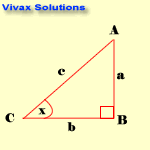• sin x = opposite / hypotenuse = a /c
• cos x = = adjacent / hypotenuse = b / c
• tan x = opposite / adjacent = a / b

The following examples are based on this triangle.

E.g.1

In the above triangle, AB = 3cm and x = 300. Find AC and BC.
sin 30 = 3 /AC
0.5 = 3 / AC
AC = 6cm.
cos 30 = b /AC
0.8660 = b / 6
AC = 5.1cm.

E.g.2

In the above triangle, BC = 4cm and x = 600. Find AC and AB.
cos 60 = 4 /AC
0.5 = 4 / AC
AC = 8cm.
tan 60 = AB /BC
1.7 = AB / 4
AB = 6.8cm.

E.g.3

In the above triangle, AB = 3cm and BC = 5cm. Find x.
tan x = 3 / 5 = 0.6
x = tan-10.6 = 28.6
x = 28.60

#### The Sine Rule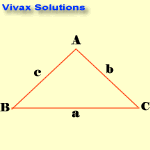sin A / a = Sin B /b = Sin C /c

Note the blinking letters to see the relationship.

E.g.1

A = 300 ; B = 500; b = 9cm; find c.
c / sin 50 = 9 / sin 30
c = 9 x sin 50 / sin 30
c = 13.7 cm.

E.g.2

A = 1100 ; b = 5cm; a = 9cm; find B.
5 / sin B = 9 / sin 110
sin B = 5*sin 110 / 9
B = 31.50

#### The Cosine Rule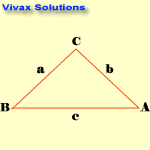c2 = a2 + b2 - 2abcosC

Note the blinking letters to see the relationship.

E.g.1

a = 4cm; b =5cm and C = 600; find c.
c2 = 42 + 52 - 2x4x5xcos60
c2 = 16 + 25 - 20
c2 = 21
c= 4.6 cm.

E.g.2

a = 5cm; b = 12cm and C = 900; find c.
c2 = 52 + 122 - 2x5x12xcos90
c2 = 25 + 144 - 0
c2 = 169
c= 13cm.

E.g.3

a = 8cm; b =7cm and C = 1200; find c.
c2 = 82 + 72 - 2x8x7xcos120
c2 = 64 + 49 + 56
c2 = 169
c= 13 cm.

E.g.4

a = 7cm; b= 6cm; c = 8 cm; find C.
82 = 72 + 62 - 2x4x5xcosC
64 = 49 + 36 - 84cosC
-84cosC = -21
cosC = 0.25
C = 75.50

#### Area of a Triangle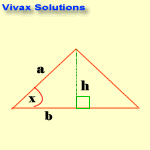A = 1/2 absinx

Proof:
A = 1/2 X h X b
sin x = h / a => h = a sinx
A = 1/2 absinx

E.g.

a = 4cm; b= 8cm; x = 300
A = 1/2 * 4 * 8 * sin 30
A = 8cm2.

#### Deriving Trigonometric Values of 00, 300, 450, 600, 900, 1800, 3600

Trigonometric values of major angles, such as 00, 900, 1800, 2700 and 3600 can be remembered with the aid of the trigonometric curves, if you can visualize them. The three major curves are as follows: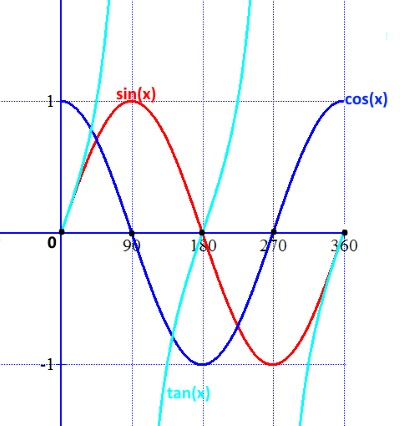The following image shows how to obtain the values of 00, 1800, and 3600 from the basic definitions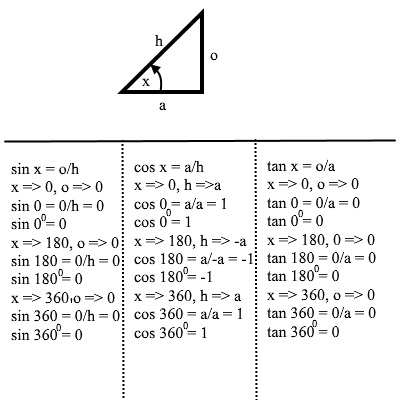The following image shows how to obtain the values of 900 and 2700 from the basic definitions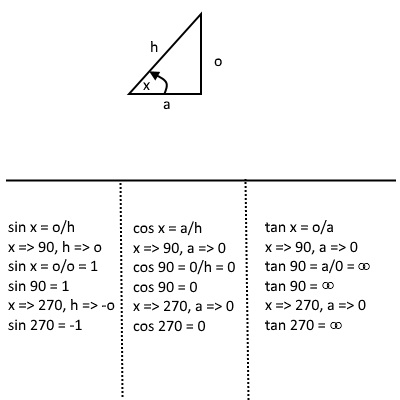In addition, the values of 300, 450 and 600 can be derived in the following way: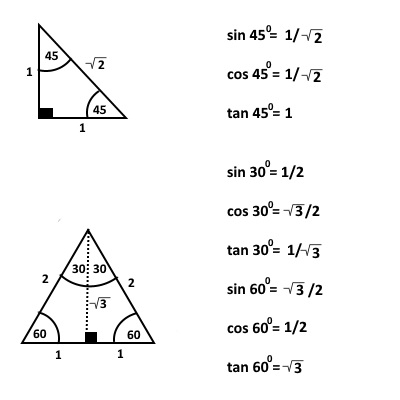The trigonometric values of 450 is calculated from an right-angled isosceles triangle with each equal side being 1 unit.
The values of 300 and 600 are calculated from an equilateral triangle of each side 2 unit.

#### Trigonometric Values in the Four Quadrants

The angles are measured from the border between the first and the fourth quadrants - and anticlockwise, by convention. If it is measured in clockwise, it is considered as negative.

The following animation explicitly demonstrates it: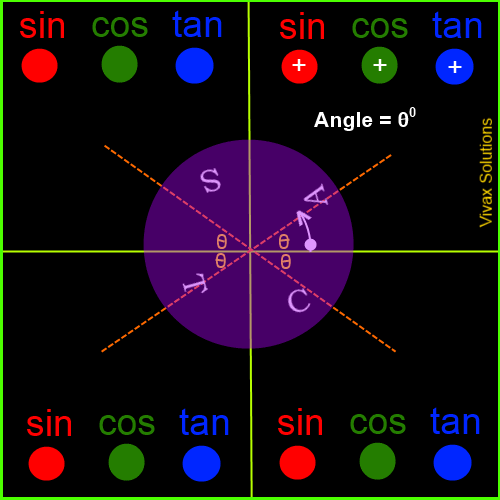Now, in order to complement what you have just learnt, work out the following questions:

1. ABC is a triangle. AB = 6cm and angle ABC = 450. Find the perimeter and the area of the triangle.
2. Prove that Pythagoras Theorem is correct using the Cosine Rule.
3. The height of a man is 1.2m. When he looks at the top of a tree, standing 20m away from it, the angle of elevation is 320. Calculate the height of the tree.
4. Points P and Q are due south and West of a pillar of height 20m. The angle of elevation of the top from these points are 220 and 420 respectively. Find the distance between P and Q.
5. A ship is sailing a distance of 200 miles from port X to port Y, on a bearing 0200. At Y, it changes its course on a bearing 1100 and reaches port Z, after travelling 360 miles. Find the distance between port X and Port Z. Find the bearing of Z from X as well.
6. A jet is flying a distance of 120 miles from town P to town Q, on a bearing 0300. At Q, it changes its course on a bearing 600 and reaches town R, after travelling 240 miles. Find the distance between town P and town R. Find the bearing of R from P as well.
7. A man is looking down from a cliff at another man on the beach. The angle of depression is 200. The second man walks 200m away from the cliff and the new angle of depression for the man at the top becomes 150. Find the vertical height of the cliff.
8. The two sides of a right-angled triangle are 5cm, 12cm. Find its size of hypotenuse and the rest of the angles of the triangle.
9. The three sides of a triangle are 8cm, 7cm and 13cm. Find its area. Calculate the shortest distance between a vertex and the longest side as well.
10. ABC is a triangle with AB = 4cm and AC = 5cm. AD is at an right angle to BC. AD = 3cm. Find BC.Maths is challenging; so is finding the right book. K A Stroud, in this book, cleverly managed to make all the major topics crystal clear with plenty of examples; popularity of the book speak for itself - 7th edition in print.

### Recommended - GCSE & iGCSEThis is the best book available for the new GCSE(9-1) specification and iGCSE: there are plenty of worked examples; a really good collection of problems for practising; every single topic is adequately covered; the topics are organized in a logical order.

### Recommended for A LevelThis is the best book that can be recommended for the new A Level - Edexcel board: it covers every single topic in detail;lots of worked examples; ample problems for practising; beautifully and clearly presented.